# Know Your Data with Continuous Probability Distribution

January 30, 2023
Stats & scoringFrom modeling the rate of radioactive decay, or the speed of sound waves to understanding the range of height, weight, blood pressure, or cholesterol level, continuous probability distributions come in handy to understand your data.

## Normal? Exponential? Uniform? Dear Data, Which One Are You?

Oftentimes we hear that data follows a normal distribution, or an exponential distribution, or a uniform distribution, and so on. Have you ever wondered what these distributions mean and why it’s important to know what exact distribution your data follows? Knowing the distribution of our data helps us gain a better understanding of the world around us. Most real-world phenomena are statistical in nature, and this applies to a broad spectrum of fields of research, from physics, economics, and finance to sociology, medicine, and engineering.

For data scientists, caring about the distribution of data influences the choice of appropriate statistical tests, gives an idea of all possible values and how often they occur, and allows to transform the actual data distribution to any of the notable data distributions in order to significantly improve the efficiency of ML algorithms ‒ proper and faster convergence and better predictions.

Probability distributions can be continuous or discrete. Rolling a die or picking a card has finite outcomes and, hence, data are said to follow a discrete probability distribution. On the other hand, a person’s height or blood pressure levels can take any value in a continuum of outcomes, so in this case, data are said to follow a continuous probability distribution.

This blog post is the first of two posts about continuous probability distribution. In this first article, Part 1 looks at the theory behind 8 common continuous probability distributions and in Part 2, we build a KNIME workflow to guess the top 5 distributions our data might be following.

Takeaways:

• Get a theoretical background of 8 common continuous probability distributions and explore how they are represented graphically

### Continuous Probability Distributions in a Nutshell

A continuous probability distribution is one in which a continuous random variable X can take on any value within a given range of values ‒ which can be infinite, and therefore uncountable. For example, time is infinite because you could count from 0 to a billion seconds, a trillion seconds, and so on, forever.

Because there are infinite values that X could assume, continuous probability distributions are expressed with a formula ‒ a Probability Density Function (PDF) ‒ describing the shape of the distribution.

Furthermore, it’s worth stressing that in continuous probability distributions the probability of X taking on any one specific value is 0. Suppose, for example, that we have a continuous probability distribution for women's weight. What is the probability that a woman will have a height of exactly 60 kg? The distribution chart will show that the average woman has a weight of 60 kg but it’s impossible to figure out the probability of any one woman weighing exactly 60 kg because the odds of it happening are so tiny that it is practically zero. She could be 60.5 kg or 59.8 kg. Because it’s impossible to exactly measure any variable that is on a continuous scale, it’s equally impossible to determine the probability of one exact measurement occurring in a continuous probability distribution.

### 8 Common Types of Continuous Probability Distribution

In this section we will go over the theoretical definition and graphical visualization of 8 common continuous probability distributions (i.e., normal, t, uniform, exponential, f, chi-square, beta, and Weibull), look at examples, and discuss why each distribution is important.

#### 1. Normal Distribution

Normal distribution, also known as Gaussian distribution, is a type of continuous probability distribution often used to approximate many real-world phenomena such as height, weight, test scores, and so on. In a normal probability distribution, most of the observations cluster around the central peak, whereas values further away from the mean taper away symmetrically on both sides and are less likely to occur.

The area under the normal distribution curve represents probability and sums to one. The normal distribution is symmetric and often called the “bell curve” because the graph of its probability density looks like a bell. The only two parameters to describe a normal distribution are the mean and standard deviation. When these parameters change, the shape of the distribution also changes (see Fig. 1). For a perfectly normal distribution the mean, median, and mode will be the same value, visually represented by the peak of the curve.Fig. 1. Normal distribution PDF’s with mean and standard deviation (Image: Normal distribution).

Among the key characteristics of a normal distribution it is worth stressing that it is unimodal i.e., it has only one high point (peak) or maximum, and its tails are asymptotic which means that they approach but never quite insect with the x-axis. This is important because it means that it is possible even very extreme values to occur by chance, at least in theory.

The formula of the PDF to calculate a normal distribution is given below:f(x) is the probability density function

x is the value or variable of the data that is examined

μ is the mean

σ is the standard deviation

When standardizing a normal distribution, the mean is fixed to 0 and the standard deviation is fixed to 1. The standard normal distribution, also called z-distribution, is a special form of normal distribution. Any normal distribution can be converted to a standard normal distribution using the formula:

z = (x - μ) / σFig. 2. Standard normal distribution (Image: Standard normal distribution).

You may be wondering why the normal distributions are so popular.

Some of the credit goes to the Central Limit theorem. The central limit theorem states that the distribution of sample means approximates a normal distribution as the sample size gets larger, regardless of the population's distribution. Moreover, contributing to the popularity of normal distributions is the simplicity to work with them since it requires only two parameters to describe the distribution. Almost all of the inferential statistics, such as t-tests, ANOVA, simple regression, and multiple regression rely on the assumption of normality.

Even though normal distributions can often be the star of the show, assuming normality has its consequences, especially when working with predictive models. For example, during error analysis, if the errors are not truly random, then this assumption will not be valid. Hence, it is always advisable to test and find out what distribution your data follow instead of just blindly going with the popular guy!

#### 2. t-Distribution

t-distribution, also known as student’s t-distribution, is a kind of distribution that looks almost identical to the normal distribution curve. Indeed, the graph is symmetric and resembles a bell-shaped curve but thicker in the tails indicating that this distribution is more prone to producing values that fall far from its mean. The t-distribution is preferred instead of the normal distribution if the sample size is small and the population's standard deviation is unknown.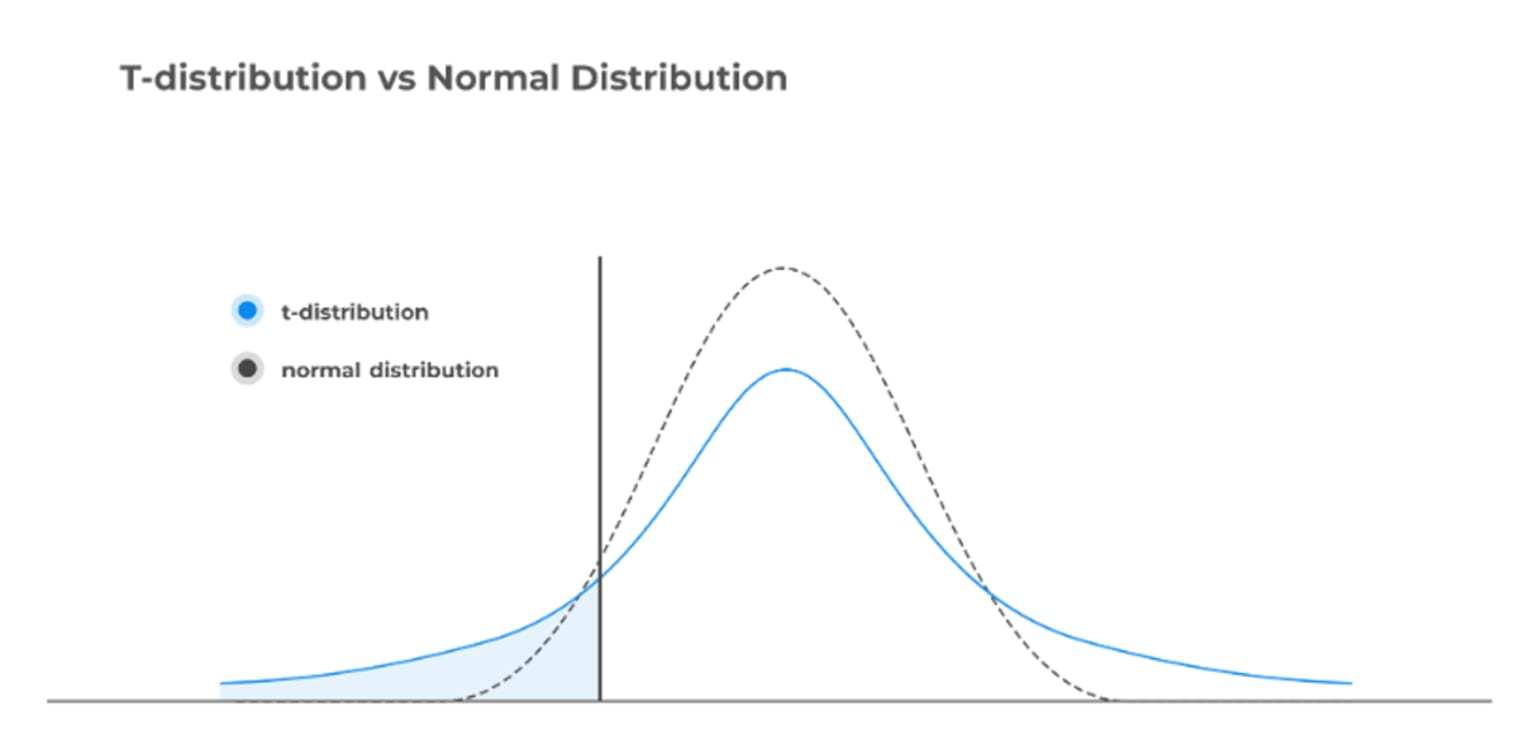Fig. 3. t-distribution vs normal distribution (Image: t-distribution).

To better understand when the t-distribution can be used, let’s consider a small example. Suppose that we want to explore the distribution of the total number of cars sold by a dealer in a month. In this case, we will use the normal distribution. Whereas, if we are dealing with the total amount of cars sold in a day, i.e., a smaller sample, we can use the t-distribution.

The t-distribution has only one parameter – the degree of freedom (). With n as the sample size, the degrees of freedom refer to the number of independent observations and can be computed by the formula:

v = n-1

With a sample of 8 observations and using the t-distribution, we would have 7 degrees of freedom. In Fig. 4 we see that as the degrees of freedom increase, the t-distribution resembles the normal distribution with the tails becoming thinner and the peaks taller.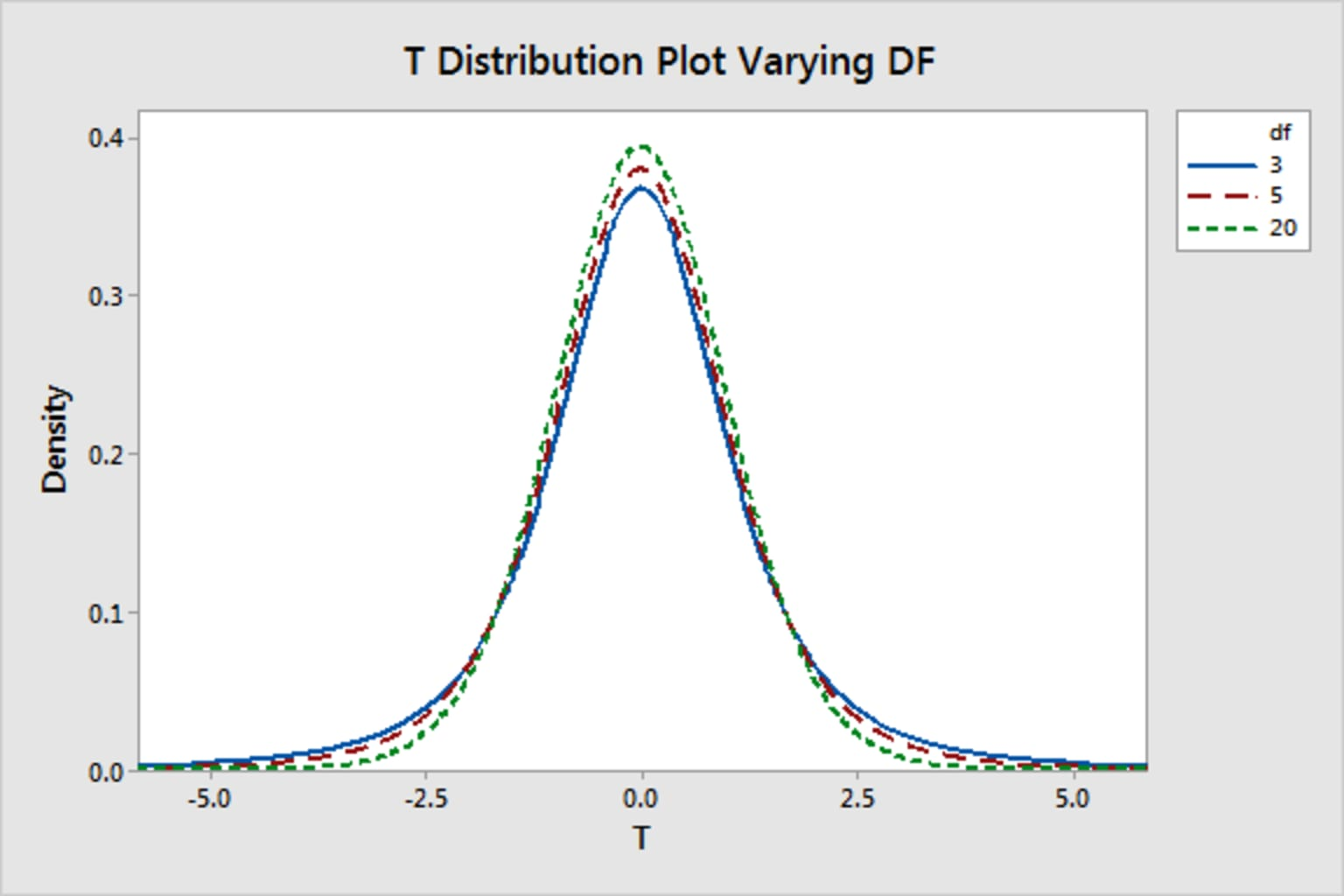Fig. 4. t-distribution with varying degrees of freedom (Image: t-distribution).

The formula of the PDF to compute the t-distribution is provided below:Where are the degrees of freedom and Γ( · ) is the Gamma function. The result y is the probability of observing a particular value of x from the t-distribution with degrees of freedom.

Notice that in the absence of explicit normality of a given distribution, a t-distribution may still be appropriate if the sample size is large enough for the central limit theorem to be applied. Indeed, for large sample sizes (>30 observations), the t-distribution looks like the normal distribution and is considered approximately normal.

The t-distribution is often used to find the critical values for a confidence interval when the data is approximately normally distributed. It is also useful in finding the corresponding p-value from a statistical test such as t-tests or regression analysis.

#### 3. Uniform Distribution

Uniform distribution, also known as rectangular distribution because of its shape (Fig. 5), refers to an infinite number of equally likely measurable values where the continuous random variable can take any value that lies between certain bounds. The bounds are defined by two parameters, a, and b, which are the minimum and maximum values. The interval can either be closed (e.g. [a, b]) or open (e.g. (a, b)). Therefore, the distribution is often abbreviated U(a, b), where U stands for uniform distribution. It is a symmetric probability distribution where all outcomes have an equal likelihood of occurring.Fig. 5. Continuous uniform distribution (Image: Continuous uniform distribution).

To understand this distribution, let’s see an example. Imagine you live in a building that has an elevator that takes you to your floor. From experience, you know that once you push the button to call the elevator, it takes between 10 and 30 seconds (the lower and upper bounds, respectively) for you to arrive at your floor. This means the elevator arrival is uniformly distributed between 10 and 30 seconds once you hit the button.

The PDF of the uniform distribution is:f(x) is the probability density function and is constant over the possible values of x.

a is the lower bound

b is the upper bound

#### 4. Exponential Distribution

Exponential distribution is used to model the time elapsed before a given event occurs. It describes the time between events in a Poisson process, i.e., the process in which events occur continuously and independently at a constant average rate. This distribution is frequently used to provide probabilistic answers to questions concerning, for example, the time associated with receiving a defective part on an assembly line, the time elapsed before an earthquake occurs in a given region, or the waiting time before a new customer enters a shop.

The key parameter of the exponential distribution is λ which is known as the rate parameter. The rate parameter indicates how quickly the decay of the exponential function occurs. Changing the decay parameter affects how fast the probability distribution converges to zero. As λ increases, the distribution decays faster, i.e, we obtain a steeper curve. We can see this in the case where λ is equivalent to 1 (Fig. 6). Decreasing the λ value translates into slower decay and when λ is close to 0 (0.1 or 0.2), the decay is minimal. Figure 6 shows the graph of an exponential distribution for different values of lambda:Fig. 6. Exponential distribution (Image: Exponential distribution).

The PDF of the exponential distribution is given below:f(x; λ) is the probability density function

x is the random variable

λ is the rate of the distribution

One important property of the exponential distribution is the memoryless property. This property helps understand the average behavior of exponentially distributed events occurring one after the other. According to this property, the past has no significance on the distribution’s future behavior. That is, irrespective of how much time has already elapsed, every instance is a new beginning. Because of the memoryless property, while observing a number of events in succession with exponentially distributed interarrival times, the expected time until the next event is always 1/λ, no matter how long we have been waiting for a new arrival to occur.

Note. It’s worth keeping in mind that the exponential distribution is related to the Poisson distribution. Suppose that an event can occur more than once and the time elapsed between two successive occurrences is exponentially distributed and independent of previous occurrences (memoryless property), then the number of occurrences of the event within a given unit of time has a Poisson distribution.

#### 5. Chi-Square (X2) Distribution

Chi-square (X2) distribution is widely used in inferential statistics for the construction of confidence intervals and in hypothesis testing. The shape of a chi-square distribution is determined by the parameter k, which represents the degrees of freedom. Similar to the t-distribution, the chi-square distribution is closely related to the standard normal distribution. This is because if we sample from n independent standard normal distributions and then square and sum the values, we will obtain a chi-square distribution with k degrees of freedom. It is noteworthy to mention that the values in the chi-square distribution are always greater than zero since all negative values are squared.

The mean of the distribution is equivalent to its k, and the variance is equal to two times the number of k. As k increases, the distribution looks more and more similar to a normal distribution. When k is 90 or greater, a normal distribution is a good approximation of the chi-square distribution (Fig. 7).

The graph of a Chi-square distribution looks as below: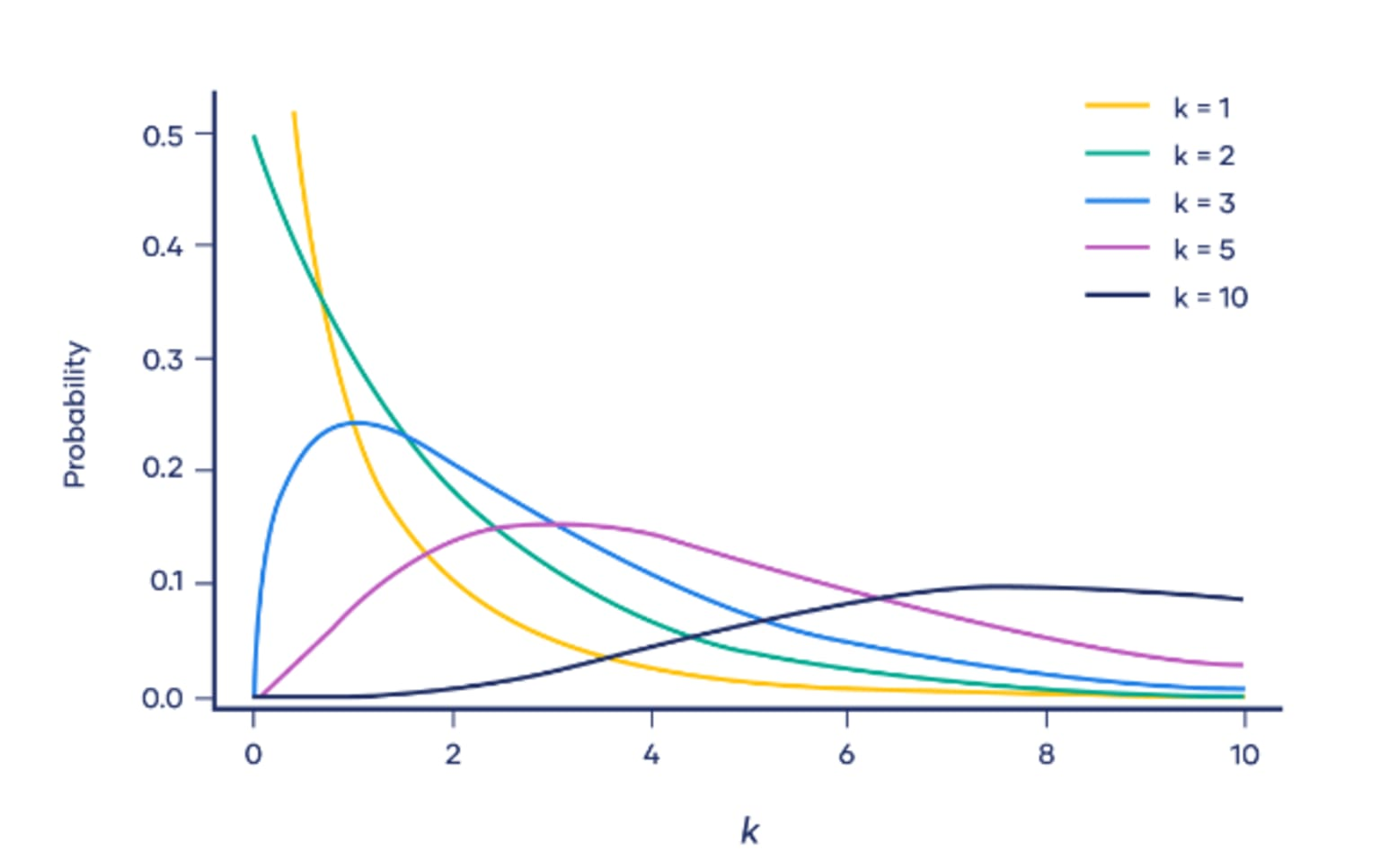Fig. 7. Chi-square distribution (Image: Chi-square distribution).

The PDF of the chi-square distribution is given below:Where f(x;k) is the probability density function, k is the degrees of freedom, and 𝚪(k/2) denotes the gamma function.

Pearson’s chi-square test is one of the common applications of the chi-square distribution. It is applied to categorical data and is used to determine whether it is significantly different from what we expected. The two major Pearson’s chi-square tests are the chi-square goodness of fit and the chi-square test of independence.

#### 6. F-Distribution

F-distribution is used for hypothesis testing and it often arises as the null distribution of a test statistic, mainly in the analysis of variance (ANOVA) to determine if the variance between the means of two populations significantly differs, or in regression analysis to compare the fit of different models. The F-distribution is closely related to the chi-square distribution but has two different types of degrees of freedom which are its key parameters. Consider two independent random variables S1 and S2 following chi-square distribution with respective degrees of freedom d1 and d2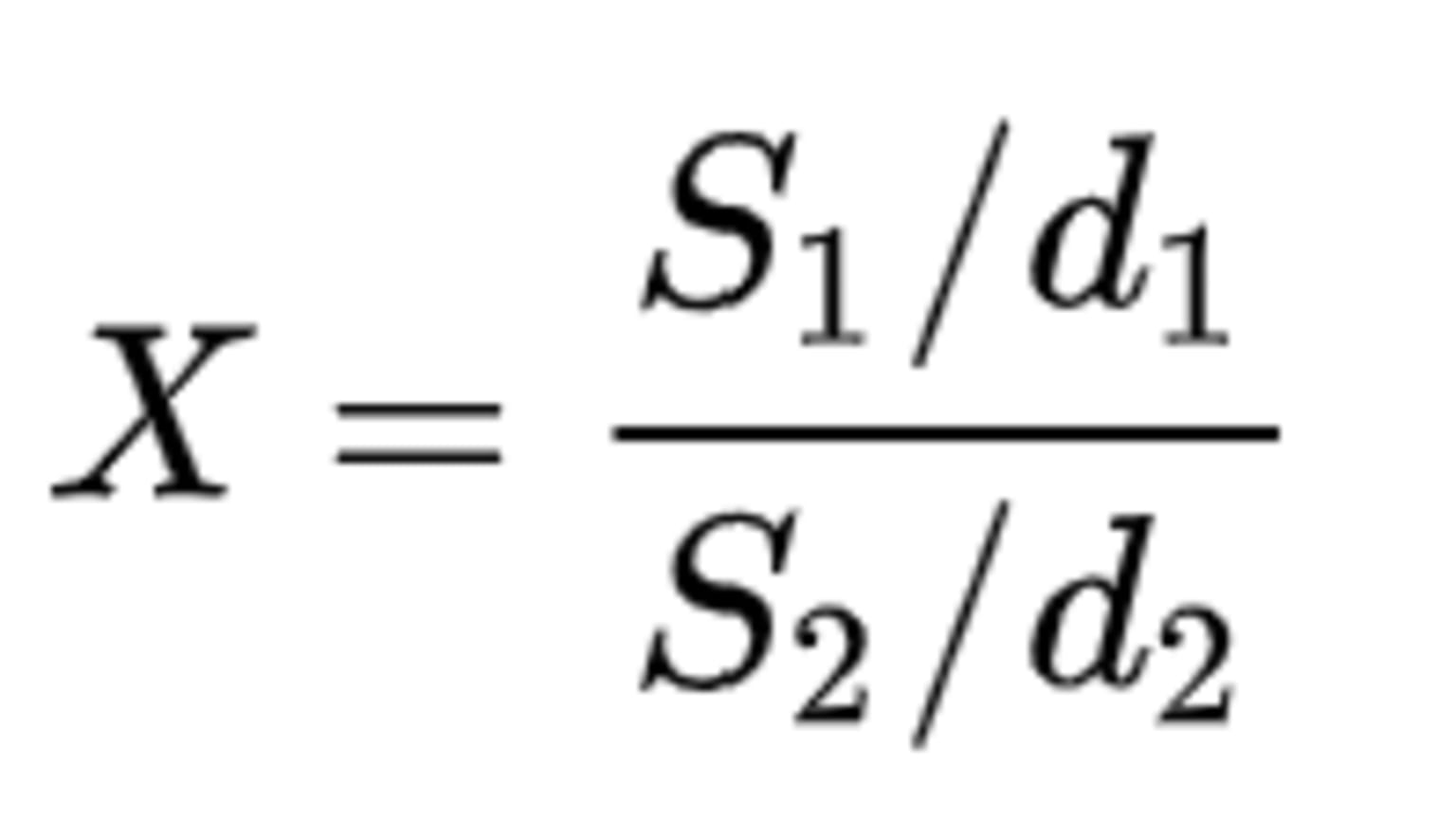The distribution of the ratio Х is called F-distribution with d1 and d2 degrees of freedom (> 0). The two degrees of freedom determine the shape of the distribution. The F-curve is non-symmetrical and is often right-skewed and starts at 0 on the horizontal axis and extends indefinitely to the right, approaching, but never touching the horizontal axis. When the degrees of freedom on the numerator are increased, the right-skewness is decreased.

Fig. 8 shows the graph of an F-distribution for different values of degree of freedom d1 and d2:Fig. 8. F-distribution (Image: F-distribution).

The PDF of the F-distribution is given below:Where f(x;d1, d2) is the probability density function of F-distribution, x > 0, B is the Beta function, and the two parameters d1 and d2 which are positive integers.

#### 7. Beta Distribution

Beta distribution models random variables with values falling inside a finite interval. The standard beta distribution usually uses the interval [0, 1] –other intervals are also possible– parameterized by two shape parameters, denoted by alpha (α) and beta (β), that appear as exponents of the random variable and control the shape of the distribution. These two parameters must be positive.

The beta distribution is closely related to a discrete probability distribution counterpart, the binomial distribution. The binomial distribution models the number of successes x with a specific number of trials while the beta distribution models the likelihood of success along with uncertainty. That is, in the binomial distribution, the probability is taken as a parameter whereas, in the beta distribution, the probability is a random variable. The beta distribution has been applied to model the behavior of random variables limited to intervals of finite length in a wide variety of disciplines.

The graph of a Beta distribution looks as below:Fig. 9. Beta distribution (Image: Beta distribution).

The PDF of the beta distribution is given below:Where f(x;α,β) is the probability density function, Β is the Beta function which acts as a normalizing constant, and α and β are the two positive shape parameters. The beta distribution can take on several shapes including bell-shaped unimodal (when α, β >1), bimodal (when 0

An intuitive example where the beta distribution comes in handy pertains to the batting averages of baseball players. The batting average is a statistic that measures the performance of batters. The beta distribution is best-suited because it allows us to represent prior expectations about most batting averages. That is, in the case where we don't know what a probability is in advance, but we have some reasonable guesses. Through beta distribution, we can represent what we can roughly expect a player's batting average to be before his first swing.

#### 8. Weibull Distribution

The Weibull distribution is another two-parameter distribution that depends on scale (λ) and shape (k), both > 0. Depending on the value these parameters take, it resembles a normal distribution or asymmetric distribution, such as the exponential distribution. It is often used for statistical modeling of wind speeds or for describing the durability and failure frequency of electronic components or materials. Unlike an exponential distribution, it takes into account the history of an object, has memory, and takes into account the aging of a component not only over time but depending on its use. It can be adapted to rising, constant, and falling failure rates of technical systems.

The graph of a Weibull distribution looks as below: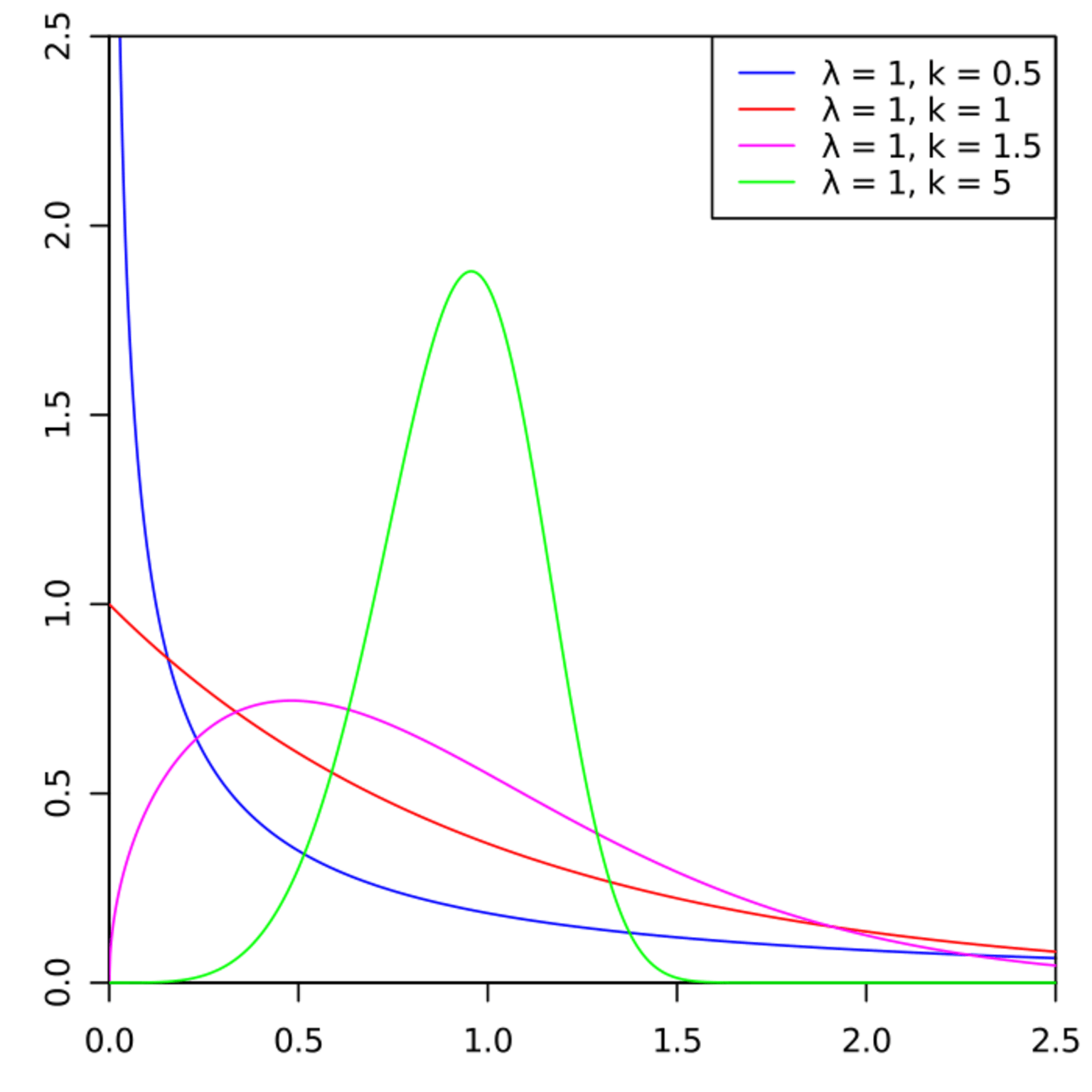Fig. 10. Weibull distribution (Image: Weibull Distribution).

The PDF of the Weibull distribution is given below: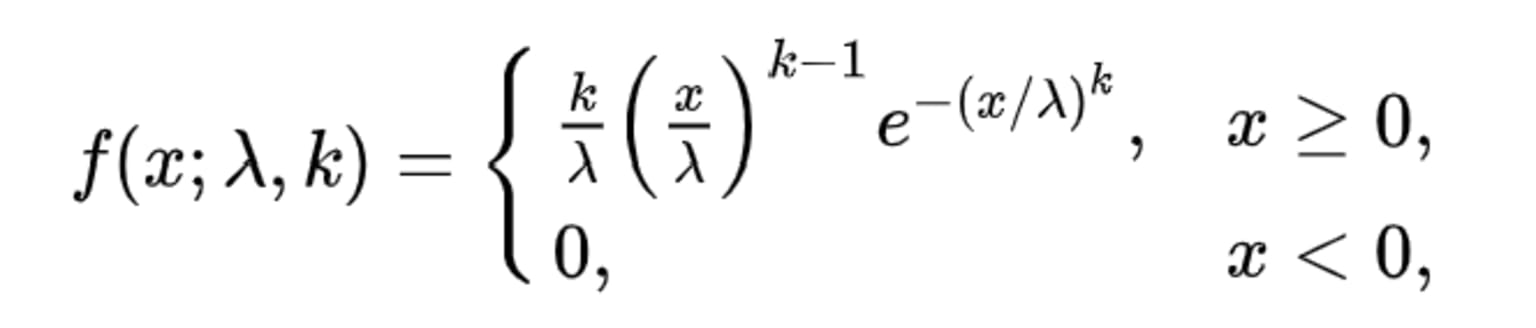Where f(x;λ,k) is the probability density function of the Weibull distribution, k is the shape parameter and λ is the scale parameter. If λ increases, while k is kept the same, the distribution gets stretched out to the right, and its height decreases while maintaining its shape. If λ decreases, while k is kept the same, the distribution gets pushed in towards the left (i.e., towards its beginning or towards 0), and its height increases. If the value of λ is kept the same while the value k=1, it leads to the exponential distribution. If the value of k1 then it has a density that approaches 0 as x approaches 1.

### Know the Distribution of Your Data with a KNIME Component

We've seen the importance to know what probability distribution your data follows before conducting any statistical tests and gone through 8 common continuous probability distribution types.

In our next article, we'll walk through the KNIME component that automatically analyzes your data to give you comprehensive information about the distribution it follows, in Identify Continuous Probability Distributions with KNIME. Download the Continuous Probability workflow from the Statistics with KNIME space on the Hub and try it out yourself.